# Biplot of PCA in R (Examples)

In this article, you will learn how to draw a biplot of a Principal Component Analysis (PCA) in the R programming language.

The table of content looks as follows:

This page was created in collaboration with Paula Villasante Soriano and Cansu Kebabci. Please have a look at Paula’s and Cansu’s author pages to get further information about their academic backgrounds and the other articles they have written for Statistics Globe.

Let’s take a look!

First of all, we will use the factoextra package. If you haven’t installed it yet, now is the right time to do it.

`install.packages("factoextra")`

The next step (or the first step if you have already installed this package) is to load the library.

`library("factoextra")`

Now, we will load our data. For this tutorial, we will use the iris dataset, which contains information about the measurements of sepal length, sepal width, petal length, and petal width from 3 species of iris and 50 flowers.

`data(iris)`

Now let’s check the number of columns and rows, and the overview of the first few rows, as shown below.

```dim(iris)
#  150   5

#   Sepal.Length Sepal.Width Petal.Length Petal.Width Species
# 1          5.1         3.5          1.4         0.2  setosa
# 2          4.9         3.0          1.4         0.2  setosa
# 3          4.7         3.2          1.3         0.2  setosa
# 4          4.6         3.1          1.5         0.2  setosa
# 5          5.0         3.6          1.4         0.2  setosa
# 6          5.4         3.9          1.7         0.4  setosa```

All is set, time to start the analysis!

## PCA

Since PCA is designed for continuous variables, we will perform our PCA for the numerical variables, excluding the “species” column. If you are interested in PCAs using categorical variables, check our tutorial: Can PCA be Used for Categorical Variables? First, we need to call the prcomp() function to run the analysis.

```pca <- prcomp(iris[, -5],
scale = TRUE)```

We have specified `scale = TRUE` inside the function to conduct a PCA using a correlation matrix, which ensures that the sensitivity to larger variable variations is taken into account.

Using the command `pca\$rotation`, we can see the loadings, which are represented by the vectors in biplots.

```pca\$rotation
#                     PC1         PC2        PC3        PC4
# Sepal.Length  0.5210659 -0.37741762  0.7195664  0.2612863
# Sepal.Width  -0.2693474 -0.92329566 -0.2443818 -0.1235096
# Petal.Length  0.5804131 -0.02449161 -0.1421264 -0.8014492
# Petal.Width   0.5648565 -0.06694199 -0.6342727  0.5235971```

We can also check the principal component scores stored in `x`, which are shown by the scatter points in biplots.

```head(pca\$x)
#           PC1        PC2         PC3          PC4
# [1,] -2.257141 -0.4784238  0.12727962  0.024087508
# [2,] -2.074013  0.6718827  0.23382552  0.102662845
# [3,] -2.356335  0.3407664 -0.04405390  0.028282305
# [4,] -2.291707  0.5953999 -0.09098530 -0.065735340
# [5,] -2.381863 -0.6446757 -0.01568565 -0.035802870
# [6,] -2.068701 -1.4842053 -0.02687825  0.006586116```

In the next sections, we will plot some biplots using the data discussed above. We don’t need to extract the data as previously shown to plot the biplots. However, if you are interested in having the exact numeric values, you can use the shared scripts above.

## Example 1: Biplot of PCA Using base R

To create a biplot using base R, we need to call the biplot() function, specifying the `pca` object and `scale = 0` since we need to scale the scores and loadings to fit the data on the same plot. For the other scaling options, see the documentation of the biplot() function.

```biplot(pca,
scale = 0)```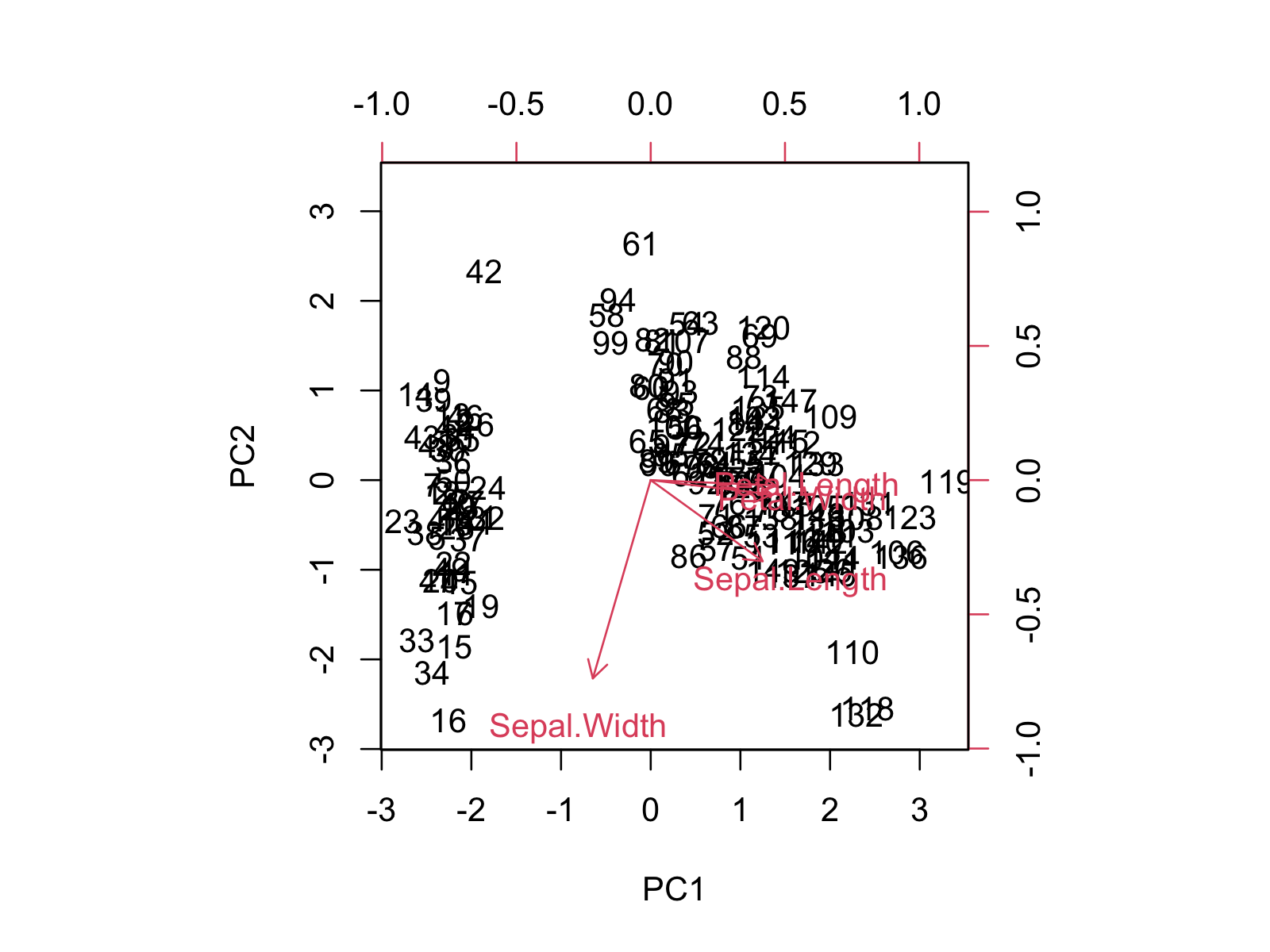The biplot shows the distribution of data points and variables concerning the first and second principal components. If you want to learn more about how to interpret the biplot, you can check our tutorial Biplot for PCA Explained.

Alternatively, we can change the color of the loading vectors and the scatter points using the `col` argument and remove the labels of the data points using the `xlabs` argument. In the rep() function, 150 refers to the number of data points, and * refers to the marker type.

```biplot(pca,
col = c('darkblue', 'red'),
scale = 0, xlabs = rep("*", 150))```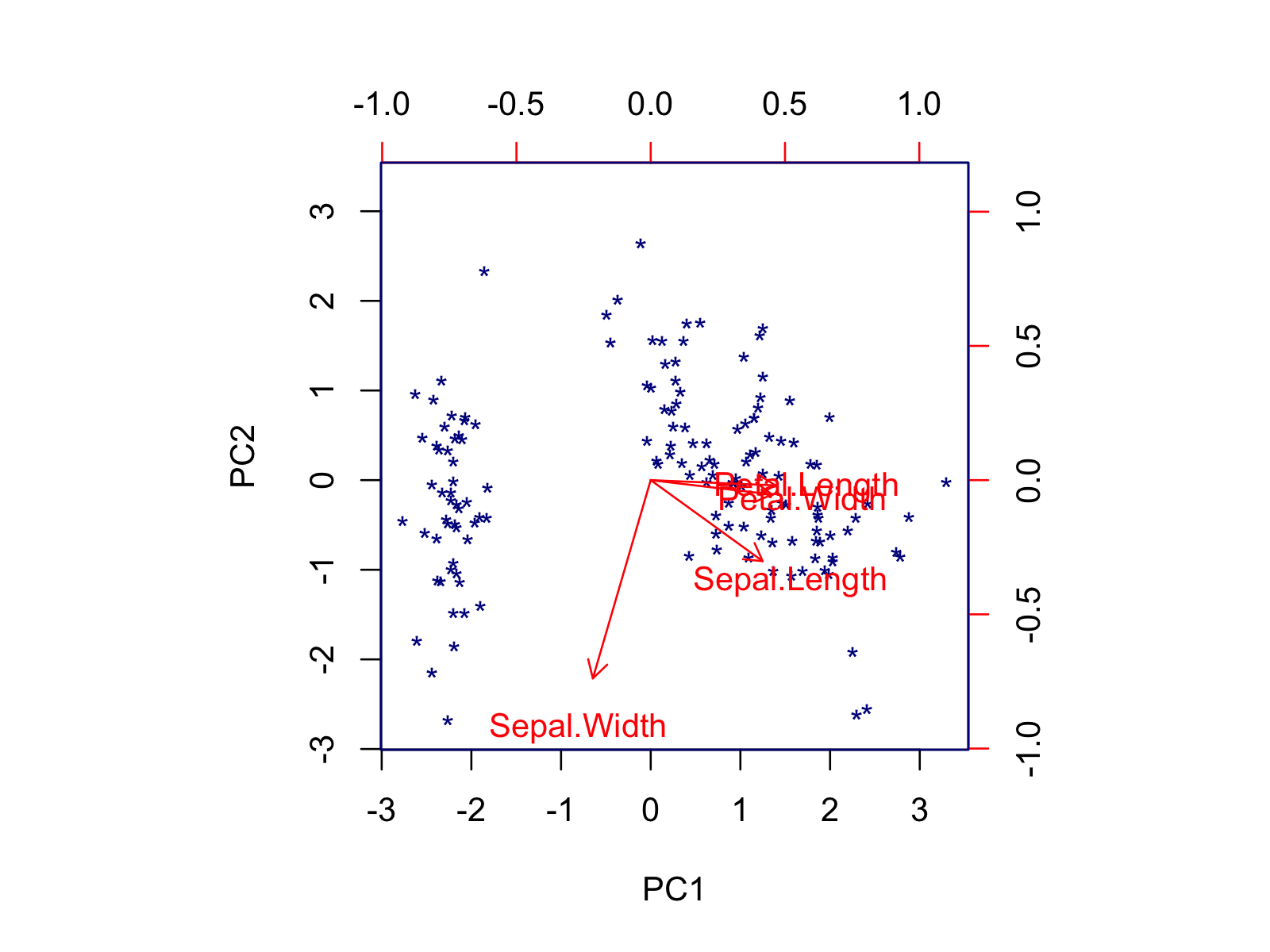Using the biplot() function, you can also modify the font size, axis limits, or length of arrows via the `cex`, `xlim`, and `expand` arguments, respectively. If you want to customize your biplot in a more advanced way, you should use the factoextra package. In the next section, some alternatives are shown.

## Example 2: Biplot of PCA Using factoextra Package

It’s also possible to create a biplot using the fviz_pca_biplot() function of the factoextra package, which is specialized to visualize PCA output. Like in base R, we must input the initialized `pca` object to run the function. Please note that we don’t specify a scaling parameter, as the scaling is done by default.

`fviz_pca_biplot(pca)`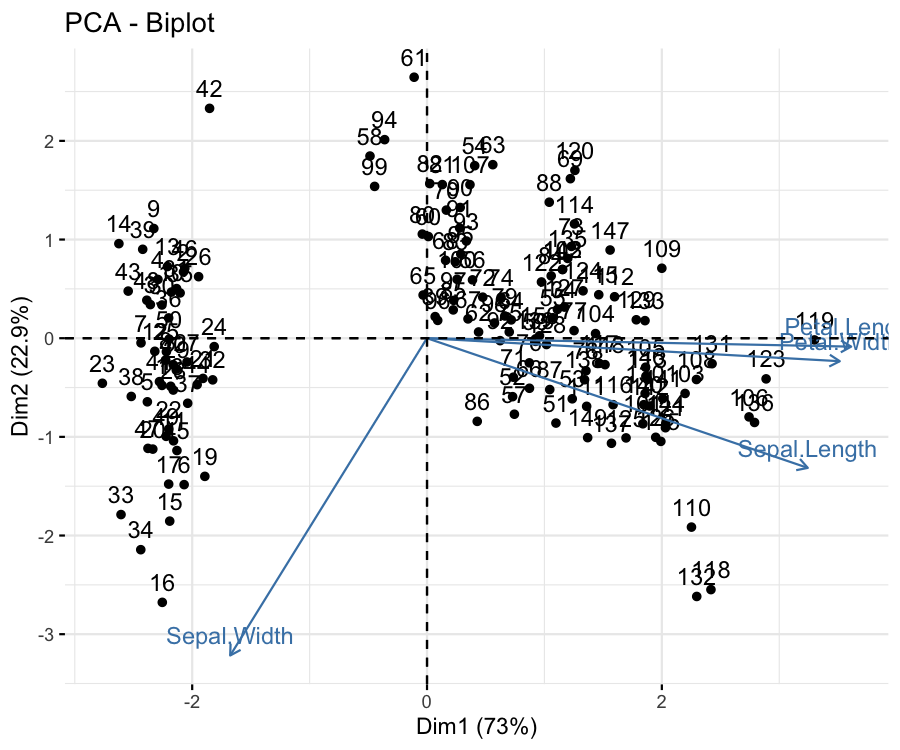The data points and the loading vectors are labeled by default, like in base R. However, different from base R, the grid lines and title are plotted, and the explained percentage of variance is shown in the axis labels.

Please be aware that the principal components are called as dimensions in factoextra, e.g., Dim1, Dim2. The labels can be modified by the labs function of ggplot2. You can also use other ggplot2 functions by appending them using the `+` operator.

We can customize the default output in different ways. For instance, we can keep only the vector labels by using `label = "var"` and color the data points by the specie using the `habillage` argument. Note that this argument also automatically changes the marker type per specie.

```fviz_pca_biplot(pca,
label="var",
habillage = iris\$Species)```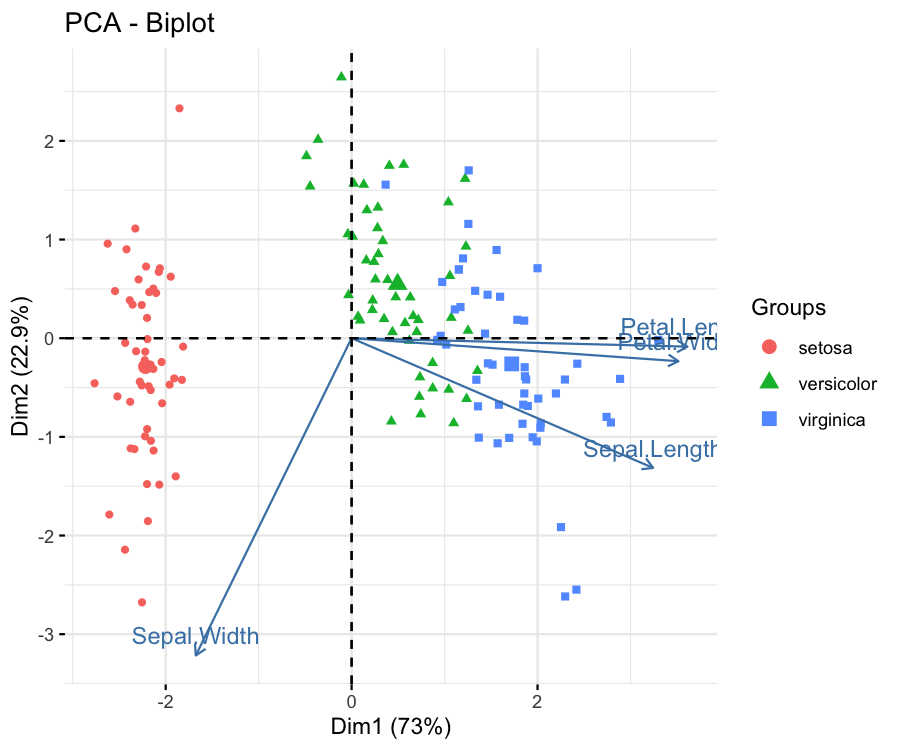The group coloring can be changed using the scale_color_manual() function of ggplot2 by setting the `values` argument to a vector of colors or using the scale_color_brewer() function of ggplot2 by specifying the color palette via the `palette`argument. If you are also interested in framing the data points by group, you can visit our Draw Ellipse Plot for Groups in PCA in R tutorial.

One might also be interested in coloring the data points based on their quality of representation by the first two components. In such a case, the `col.ind` argument should be set to `"cos2"`, as shown below. The default colors can be changed using the argument `gradient.cols` and the vector colors can be set to a unique black color via `col.var = "black"`.

```fviz_pca_biplot(pca,
label = "var",
col.ind = "cos2",
col.var = "black",The figure shows that the flower samples are represented well if they are colored red and poorly represented if colored blue. The same coloring can also be set for the loading vectors via `col.var = "cos2"`.

Another way of coloring by statistics is coloring by the contribution to the components. See below how the variable’s contributions are shown through the colors of loading vectors by setting `col.var = "contrib"`.

```fviz_pca_biplot(pca,
label = "var",
col.ind = "black",
col.var = "contrib",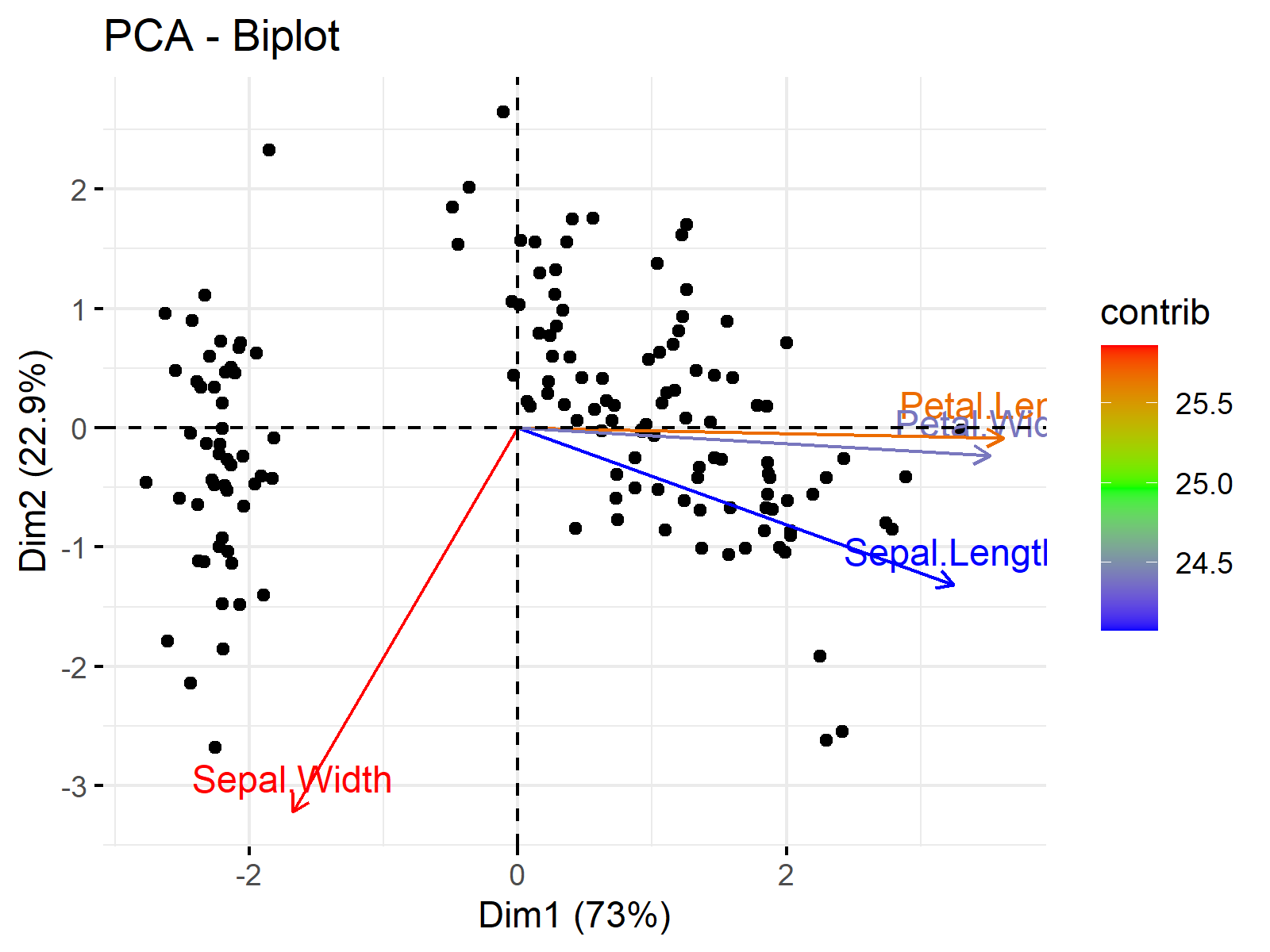This is the end of our tutorial explaining how to plot biplots in R. We have shown the options provided by the base and factoextra libraries. Alternatively, the autoplot() function of ggfortify, which is a more generic function to visualize multivariate data, can be used for plotting biplots. If interested, see Autoplot of PCA in R.

## Video, Further Resources & Summary

Do you want to know more about performing a PCA in R? Then you should have a look at the following YouTube video of the Statistics Globe YouTube channel.

Please accept YouTube cookies to play this video. By accepting you will be accessing content from YouTube, a service provided by an external third party.If you accept this notice, your choice will be saved and the page will refresh.

In addition, you may want to have a look at some of the other tutorials on Statistics Globe:

In this post you have learned two examples of how to make a biplot of a PCA in R. Leave a comment if you have any questions.

Subscribe to the Statistics Globe Newsletter

•Alexandre
May 16, 2023 6:38 pm

Dear all,
Can someone help me?
I would like to format the biplot variables in different ways. For example, the names Sepal.Width and Sepal.Length wanted the first to be Sepal subscribed Width and the other Sepal.Length in italics.
Yours sincerely,
Alexandre Jardim.

•May 19, 2023 8:46 am

Hello Alexandre,

Renaming the variables is very easy using the names function. With that, you can rewrite Sepal.Width as Sepal_Width as follows.

```#rename data
data(iris)
names(iris)<-c("Sepal.Length", "Sepal_Width",  "Petal.Length", "Petal.Width",  "Species")```

Then you can perform pca. As you said, it is not trivial to label the vectors in italics since, in the factoextra package, the fviz_pca_biplot function doesn’t provide an option to change the font style of loading labels directly. However, you can do it manually using the geom_segment and geom_text functions of ggplot2.

```# load necessary libraries
library(FactoMineR)
library(factoextra)
library(ggplot2)

# perform PCA
res.pca <- PCA(iris[, -5], graph = FALSE)

# get PCA biplot without labels
biplot <- fviz_pca_biplot(res.pca, invisible="var")
biplot

# extract variable coordinates
var.coord <- get_pca_var(res.pca)\$coord
var.coord

biplot +
geom_segment(data = as.data.frame(var.coord),
aes(x = 0, y = 0, xend = 3* Dim.1, yend = 3 *Dim.2),
arrow = arrow(length = unit(0.2, "cm")), color="blue") +
geom_text(data = as.data.frame(var.coord),
aes(x = 3* Dim.1, y = 3 *Dim.2, label = rownames(var.coord)),
fontface = "italic", hjust = -0.1, vjust = 0.5)```

Please be aware that I rescaled the arrow lengths, which is done by default if you use the default settings of fviz_pca_biplot, to ensure that the arrows are visible in the graph. You can observe the visual below.Regards,
Cansu

•Alexandre
May 19, 2023 12:13 pm

Dear Cansu,
Thank you very much for your contribution.
Unfortunately, my problem has been partially resolved. I would really like my variable to be subscripted (i.e., Sepal””Width).
I tried using the “” tag but it didn’t work. Do you know of any solutions?
Regards,
Alexandre.

•May 19, 2023 2:17 pm

Hello Alexandre,

You can easily change it by using the names function as I previously shared names(data)<-c("anyformat",...). If it doesn't answer your question, could you please give some examples for me to understand? Regards, Cansu

•Enik Nurlaili Afifah
August 9, 2023 1:15 am

how to rename the data points (individual), for example, the individuals are names of varieties/species

•August 9, 2023 7:37 am